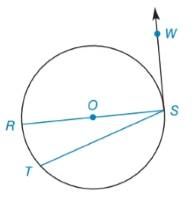Chapter 6.2, Problem 4E### Elementary Geometry for College St...

6th Edition
Daniel C. Alexander + 1 other
ISBN: 9781285195698

#### Solutions

Chapter
Section### Elementary Geometry for College St...

6th Edition
Daniel C. Alexander + 1 other
ISBN: 9781285195698
Textbook Problem
1 views

# Find m R T ⌢ if m ∠ R S T : m ∠ R S W = 1 : 5To determine

To find:

mRT if mRST:mRSW=1:5.

Explanation

Theorem:

The radius drawn to a tangent at the point of tangency is perpendicular to the tangent at that point.

Corollary:

The measure of an angle formed by a tangent and a chord drawn to the point of tangency is one-half the measure of the intercepted arc.

Calculation:

To find: mRSW.

Diameter is RS¯ and tangent is SW.

By theorem,

mRSW=90°

mRST:mRSW=1:5

mRSTmRSW=15mRSW=5mRST90°=5mRST

### Still sussing out bartleby?

Check out a sample textbook solution.

See a sample solution

#### The Solution to Your Study Problems

Bartleby provides explanations to thousands of textbook problems written by our experts, many with advanced degrees!

Get Started

#### Simplify the expressions in Exercises 97106. (xy)1/3(yx)1/3

Finite Mathematics and Applied Calculus (MindTap Course List)

#### Using the power series for cos x, the sum of the series is: cos(0.0625) cos(0.25) cos(0.5)

Study Guide for Stewart's Single Variable Calculus: Early Transcendentals, 8th

#### Define a construct and explain the role that constructs play in theories.

Research Methods for the Behavioral Sciences (MindTap Course List)